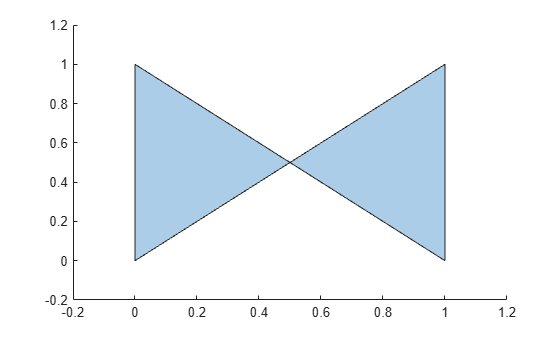# simplify

Simplify `polyshape` boundaries

## Syntax

``polyout = simplify(polyin)``
``polyout = simplify(polyin,'KeepCollinearPoints',TF)``

## Description

example

````polyout = simplify(polyin)` returns a `polyshape` object made up of the boundaries of the polygon `polyin` with all vertex duplicates removed, and all boundary intersections and improper nesting resolved.```
````polyout = simplify(polyin,'KeepCollinearPoints',TF)` specifies whether to keep or remove collinear points in `polyout`.```

## Examples

collapse all

Create and plot a polygon that contains a boundary intersection.

```P = [0 0; 1 1; 1 0; 0.5 0.5; 0 1; 0 0]; polyin = polyshape(P,'Simplify',false)```
```polyin = polyshape with properties: Vertices: [5x2 double] NumRegions: 1 NumHoles: 0 ```
`plot(polyin)`Use the `simplify` function to remove the intersection, which produces a well-defined polygon. Simplifying the polygon maintains the boundary shape, but splits the polygon into two distinct regions.

`polyout = simplify(polyin)`
```polyout = polyshape with properties: Vertices: [7x2 double] NumRegions: 2 NumHoles: 0 ```

## Input Arguments

collapse all

Input `polyshape`, specified as a scalar, vector, matrix, or multidimensional array.

Data Types: `polyshape`

Collinear vertices indicator, specified as `false` or `true`:

• `false` — Remove collinear points so that the output `polyshape` contains the fewest vertices necessary to define the boundaries.

• `true` — Keep all collinear points as vertices.

When the `'KeepCollinearPoints'` parameter is not specified, its value is automatically set to the value used when creating the input `polyshape`.

Data Types: `logical`

## Version History

Introduced in R2017b